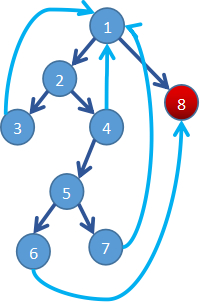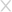## Test1

``````        int a = Math.getExponent(88);
int y = 0;
1	while(y>0){
2	    if(a<=0){
3		 a=a+1;
y=y+1;
}else{
4		 if(a>10){
5		     if(a>100){
6		         a=a*5;
break;
}else{
7			 y=y/a;
}
}
}
}
8	this.attachBaseContext(this);``````GDA看起来是最接近源代码的，且保持了语义的准确性，并且识别出了符号。

GDA
JEB × 一般

## Test2

``````        int a = Math.getExponent(88);
int y = 0;
1	while(y>0){
2	    if(a<=0){
3		 a=a+1;
y=y+1;
}else{
4		 if(a>10){
5		     if(a>100){
6		         a = a*5;
break;
}else{
7			 y = y/a;
}
}
}
8             y = y*y;
}
9	this.attachBaseContext(this);``````GDA
JEB × 一般

## Test3

``````        int a=Math.getExponent(88);
int y=0;
1	while(y>0){
2	    while(a>0){
3	        if(a<=0){
4	            a=a+1;
y=y+1;
}else{
5 	            if(a>10){
6	                 break;
}
}
}
}
7	this.attachBaseContext(this);``````GDA
JEB ×

## Test4

``````        int a=Math.getExponent(88);
int y=0;
1	while(y>0){
2	    while(a>50){
3	        a=a+1;
y=y+1;
4 	        if(a>10){
5	            if(a>100){
6		        a=a*5;
break;
}else{
7			y=y/a;
}
}
}
8           this.attachBaseContext(this);
}
``````GDA
JEB

## Test5

``````        int a=Math.getExponent(88);
int y=0;
1	while(y>0){
2           y++;
3	    while(a>50){
4	        a=a+1;
y=y+1;
5 	        if(a>10){
6	            if(a>100){
7		        a=a*5;
continue;
}else{
8			y=y/a;
}
}
9               y=a*y;
break;
}
10          this.attachBaseContext(this);
}
``````从反编译结果上来看，GDA还原代码时，虽然保持了与源码较高相似性，但是却因丢失了a=a*5语句后的continue语句导致语义错误。而JEB还原代码时，虽然保持语义的正确性，但是代码还原度比较低。Jdax和GDA一样都出现了语义错误，并且不仅丢失了continue语句而且还丢失了break语句。

GDA ×
JEB

## 结论### 相关推荐

#### 2019互联网安全城市巡回赛·北京站——巅峰对决，邀你来战！### 填写个人信息

• 可以给我们打个分吗？